#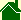The Ball of Light Particle Model

The Grand Unification Theory, The Ball-of-Light Particle Model is a new Grand Unification Theory that unifies all areas of physics. It is the first Grand Unification Theory that unifies the gravitational force with the electric and magnetic forces.

### The vector E cross the vector B equals the vector G

The Ball-of-Light Particle Model describes all elementary particles as standing, spherical waves composed of three fields: electric, magnetic, and gravitational. (The vector G is not equal to the gravitational constant G, or the lower case "g" as in F = mg.) The central equation: E cross B = G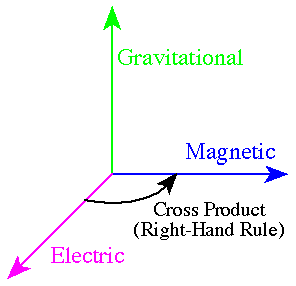emphasizes the already known fact that the electric, magnetic and gravitational force fields act at right angles to each other. (An easy way to imagine this is picture the corner where two walls meet a ceiling.) The relationship between these three fields has been known to exist and is expressed in a widely accepted physics equation known as the "Poynting Vector".

### The Poynting Vector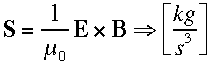The Poynting Vector "S" is expressed in terms of the Electric and Magnetic fields. However, note the units can be reduced to kilograms per second cubed! This equation expresses mass in terms of the electric and magnetic concepts. What is "new," so-to-speak, is to
integrate the cross product -- E cross B -- over the surface of a sphere to obtain mass or gravity. (This relationship can be seen in Table A.)

(This page contains information that is not in the book, "The solution to the question, "What is time?" This information has been submitted to the journal Physical Review -- in 1995 -- and is on record with that publication under the name, "Ball of Light Particle Model." Until this paper has been published with Physical Review -- or another journal -- this information will remain: copyrighted (1995) by John T. Nordberg -- all rights reserved.)

### Table A -- a Summary of The Ball of Light Particle Model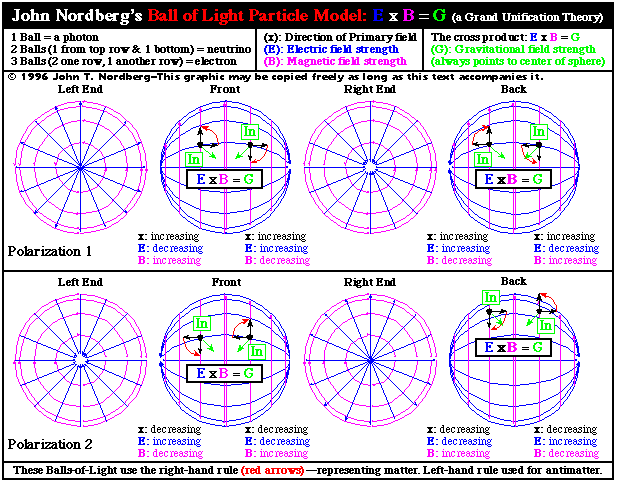### Table A Summary

Understanding this table is the most important step that someone must undertake in order to understand the Ball-of-Light Particle Model.

Details to notice include:

1. Notice how Table A has two halves. These two halves represent opposite polarizations. These two polarizations represent just one quadrant of a much larger table. The complete table -- Table B (Warning! This is a very large graphic) -- has 4 quadrants -- each with 2 polarizations.
2. The 3 fundamental fields are used to describe each of the spheres.
3. The electric field is represented by blue arrows.
4. The magnetic field is represented by magenta arrows.
5. The gravitational field is represented by green arrows.
6. Table A represents "matter" and uses the "right-hand" rule.
7. The "right-hand" rule is not physically special and thus, this table -- to be logically valid -- should also be able to be created using the "left-hand" rule. This can be seen in Table B (Warning! This is a very large graphic). Careful analysis reveals that "antimatter" is represented when these balls-of-light are expressed using the left-hand rule.
8. You have to start somewhere. I started with the second sphere from the left in the top row. It looks like this: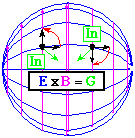9. You have to start by picking a field -- either electric, or magnetic -- to sweep over the sphere. The field picked to "sweep" over the sphere is called the "primary" field. (I picked the electric field to be the primary field based on a hunch that results in the field given off by an electron.) Note that it is sweeping from left to right in the above sphere.
10. You have to start by picking a cross-product rule -- either left-hand, or right-hand. I picked the right-hand rule.
11. You have to start with the primary field sweeping either to the left or to the right. I picked to the right.
12. You have to pick your "signage." For the "x direction" -- which is left-to-right -- I picked, if the primary field is sweeping to the right it is positive, if it is sweeping to the left it is negative. For the "y and z directions" I picked, if the "diameter" of the primary field is "expanding" then the value is negative, if it is contracting, then the value is positive. (Whether the Electrical and Magnetic fields are increasing or decreasing doesn't matter -- their values are always greater than or equal to zero.)13. With these starting points, notice how the primary field -- the electric field -- sweeps over the sphere, from the left pole to the right pole.
14. As the primary field sweeps over the sphere in the left hemisphere, the field's "density" spreads out over a greater area. In this case the electric field is decelerating.
15. The deceleration of the primary field -- in this case, an electric field -- induces a secondary field -- in this case, a magnetic field -- at a right-angle to the primary field. (There is absolutely no doubt that nature acts this way. The induction a magnetic field from changing an electrical field is a proven fact.) In Table A, in this sphere, using the right-hand rule, treating "the deceleration" as "negative", the direction of "curl" would have the induced magnetic field pointing "up" on the front of the hemisphere, and pointing "down" on the back, unseen side of the hemisphere. Graphic of hand curling
16. This can be seen better with the first sphere in the top row which represents looking at the sphere from the left end. From this end the secondary field appears to be turning counterclockwise.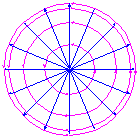(Left end of first ball of light in Table A)

17. Before continuing, I should exactly explain what I mean here by "acceleration" and "deceleration." The fields are always moving at the speed of light -- always! What is accelerating and decelerating is "field strength." As the field expands, at the speed of light, the field strength decelerates. As the field contracts, the field strength accelerates.
18. Notice as the field strength for the primary field expands and decelerates, the energy "lost" is transferred to the induced secondary field. Thus, the field strength of the secondary field accelerates in a perfectly symmetrical correspondence to the deceleration of the primary field.
19. As the primary field weakens, the secondary field strengthens. What does this do? It bends the primary field along the sphere. The secondary field changes the direction of the primary field.
20. At the equator, the situation reverses itself. The primary field has become deflected to the point where it is no longer is expanding but contracting.
21. Since the primary field is contracting on the right hemisphere, its field strength is accelerating. Again, this induces a secondary field. However, the secondary field must change direction. Viewed from the right end of the sphere, the secondary field appears to turning counterclockwise.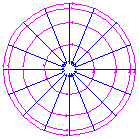(Right end of first ball of light in Table A)

22. But what about gravity? At every point on the sphere, if you analyze the cross-product of the primary and secondary field, what is the result? The cross product of E cross B = G always points to the center of the sphere!
23. Let's review signs. Examine an arbitrary point on the left hemisphere. The direction of the primary field -- and the secondary field moving with it -- is to the right, defined here as positive. Both the primary and secondary fields are expanding -- moving away from the x-axis -- defined here as negative.
24. Examining the point on the left hemisphere, using the right-hand rule, crossing E to B, would initially give the direction for the cross product pointing out. However, the sign is negative (x is positive, y and z is negative). Therefore, the direction of the cross-product is towards the center of the sphere.
25. Examining an arbitrary point on the right hemisphere, using the right-hand rule, crossing E to B, the direction would point in. The sign is positive (x is positive, y and z is positive). Therefore, the direction of the cross-product is not reversed. [If how I define my signs bothers you, it doesn't matter. After analyzing all of the possible variations, you will find the situation is perfectly symmetrical. Therefore, if my signs are all completely backwards, then all that is needed is to make the Grand Unification Formula, E cross B = -G, instead of E cross B = G. Personally, I don't care which is which -- the symmetry is perfect either way.]
26. The bottom row is an example that starts with primary field sweeping from right-to-left instead of left-to-right. This row is not equivalent to the top row. The bottom row is perfectly symmetrical with the top row, but exactly opposite in every respect except. (The cross product still points inward!) These two rows have exactly opposite "polarizations." If you have ever heard of polarization before but could not really understand what it was, then this is your answer.
27. What about using the right-hand rule? Doesn't that seem completely arbitrary to you? It does to me! I reasoned, if this is how nature really works, it shouldn't matter if you use the right-hand rule, or the left-hand rule. This can be seen in Table B (Warning! This is a very large graphic) which is the complete combination of possibilities using the Ball-of-Light Particle Model.
28. Also, what about using the electric field as primary? Doesn't that also seem completely arbitrary to you? It does to me! I reasoned, if this is how nature really works, then it shouldn't matter if you use the electric or the magnetic field as primary. This can also be seen in Table B (Warning! This is a very large graphic) which is the complete combination of possibilities using the Ball-of-Light Particle Model.

### Table B

Table A is only one fourth of a much larger graphic -- Table B. Table B is too large to make a convenient Internet graphic. To see each portion, click in the table below.

### Understanding Table B

If the Ball-of-Light Particle Model is correct, then what do the 4 quadrants of Table B represent? I believe that one quadrant represents matter, one represents antimatter, and the other 2 quadrants represent "matter" and "antimatter" in another, opposite half of the universe! Our part of the universe seems to use the electric field as the primary field and the most matter behaves with the right-hand rule. There must be another half of the universe that follows the left-hand rule. In essence, everything there has an opposite spin.

There is a lot of speculating that could be done here. However, the key thing to notice in Table B are the symmetries. They are everywhere! If the Ball-of-Light Particle Model does not describe nature, then it would be natural to ask why? My first impression was, this has to be it!

Now, back to basic rules of science. Does the Ball-of-Light Particle Model match up with any observations in physics? Yes. Particle Physics? Yes. How about astronomy? Yes. Are there any situations -- any observations -- that contradict the Ball-of-Light Particle Model? I haven't discovered one yet.

### Superposition

What should we talk about next? There are so many possibilities. What is a photon? What is a neutrino? What is an electron? Before getting to these questions, the key area, will be something called superposition. It deals with adding these balls-of-light together. In other words, what happens when one of the balls-of-light meets another ball-of-light?

Ask yourself, what would happen if two of these waves of electromagnetic and gravitational fields were combined? Some of the fields could add to each other. Some of the fields could subtract from each other.

Imagine waves in water. What happens when two waves superimpose? Their heights add. What happens if the two waves our out phase? They subtract.

Graphic of waves

In symmetrical situations, equal and opposite electrical or magnetic fields would attract each other. In nonsymmetrical situations, the fields would repulse each other, or, create a disturbance that could destroy the particle.

The gravitational fields of two spherical waves would always combine to make the two particles more stable.

Equal and identical electrical or magnetic fields would create situations where the two balls-of-light would repel each other and never perform a superposition. In other words, they could not add because they couldn't combine in a stable manner.

### Implications for physics

The Ball-of-Light Particle Model does not reject all of previous physics. It builds on it. However, some areas of previous physics must be refuted.

Relativity is the key area. It needs to be completely thrown out.

The standard model for an atomic nucleus, while not completely refuted, must be modified significantly. Every student of science has had it pounded in to them that atomic nuclei are collections of protons and neutrons held together by the strong force. The Ball-of-Light Particle Model predicts atomic nuclei are spherical balls-of-light that are held together by a strong combination of three forces: electric, magnetic, and gravitational.

The standard model for the star and galaxy formation needs to be completely redone. The Ball-of-Light Particle Model predicts they are decaying balls-of-light rather than collapsing clouds of primordial hydrogen and helium.

The standard model for cosmology, the Big Bang, also needs significant reworking. Instead of expanding from a singularity, the universe is expanding from an exploding ball-of-light.

Furthermore, I believe the Ball-of-Light Particle Model predicts that the matter and energy of the Big Bang are expanding in space, rather than space "itself" is expanding.

### Summary of what a ball of light is

Pick up a billiard ball. It seems solid. Cut it in half. It looks solid. But looks are deceiving. At the atomic level the atom is mostly void. There is a central nucleus, and it is surrounded by orbiting electrons. Thus, the billiard ball is mostly void.

graphic of atom

Can you cut an electron in half like the billiard ball? Is it solid? It is impossible to "see" an electron. We "see" by reflecting photons off objects. However,
photons can interact with electrons. Thus, there is no way to visually "see" the structure of an electron.

Can you cut a nucleus in half? In general, the answer is yes. Can you "see" its structure? In general, no. It is only possible to visualize atomic nuclei. Presently, the nuclei of atoms are visualized as
"clusters" of separate particles, called protons and neutrons, that are held together by the "strong" force -- with the exception of the nucleus of the Hydrogen atom which has just one proton. The Ball of Light Particle Model differs from this traditional view of nuclei. The Ball of Light Particle Model describes all elementary particles -- including traditional atomic nuclei and photons -- in terms of a spherical, 3-dimensional wave of Electric, Magnetic, and Gravitational fields. Since the Ball-of-Light Particle Model treats elementary particles and nuclei as being "built up" from just photons, this particle model can be summarized as describing all elementary particles as "Balls of Light." (A minor aspect of this particle model, is it predicts the centers of these balls of light are voids -- the electric and magnetic fields are just on the surface of the spheres.)

Both, the traditional particle model, and the Ball-of-Light Particle Model can never be proven. Since there is no way to see the structure of the particles described, they can only be supported by inductive reasoning.

### Photons (The first harmonic)

In Table A, in the top row of spheres, is a sphere labeled, "Front." By itself, one of these spheres represents a photon. Key aspects of this sphere are:

• The diameter of the sphere determines the photon's frequency.
• This sphere can be thought of as either a wave, or a particle.
• The Electric and Magnetic fields are moving at right angles to each other. If you multiply their speeds you get c2 ("c" squared).
• The Electric and Magnetic fields perfectly obey the laws of induction. (Now it is seen that gravity also obeys the laws of induction.)
• In this sphere, the electric field (blue) has a translational speed to the right at the speed of light. While the magnetic field (magenta) is moving at the speed of light -- it rotates around the axis of movement.
• It is known that a photon does not dissipate as it moves away from its source. This particle model explains why. The 3 forces work in harmony wrapping around themselves. The electric field induces the magnetic field. The magnetic field induces the electric field. The cross product of the electric and magnetic induces the gravitational field. The gravitational field keeps the electric and magnetic fields wrapping around a center pivot point.
• This particle model does not use "gravitons" since it treats the photon as the carrier of the gravitational field. This is why light is bent by gravitational fields -- the interaction is built-in.
• This particle model allows the superposition of multiple photons.
• These spheres represent only one fourth of a much larger table -- Table B. The spheres in Table A treat the Electric field as "primary." The "primary field" is the field that sweeps over the surface of the sphere -- from pole to pole. The secondary fields spin around the two hemispheres of the sphere accounting for angular momentum. (However, there is nothing that would prevent the magnetic field from being the primary field, and the electric field as being secondary.)
• The spheres in Table A are using the "right-hand" rule. The "left-hand" rule is equally valid. This particle model assumes that "matter" follows the "right-hand" rule, and "antimatter" follows the "left-hand" rule.
• This particle model treats photons as the "first harmonic."

To see how a photon moves, examine the following graphic.
Animation of photon moving.

### Neutrinos (The second harmonic)

Examine the two spheres in Table A labeled "Front" -- in both the top and bottom rows. Notice how they have:

• identical wavelengths
• are moving in opposite directions
• are polarized in opposite directions

This particle model hypothesizes, if these two photons are superimposed, they will no longer have "translational" motion. Their fields would interact -- creating a "standing wave." The particle that would be formed would:

• have a neutral charge
• would have a spin -- each hemisphere spinning in the opposite directions
• would be extremely stable, with little reason to interact with other particles
• would have a small net gravitational force -- while the electric and magnetic fields would neutralize each other, the gravitational fields would combine

These characteristics closely match current descriptions of neutrinos. This particle is called the "second harmonic."

### Electrons (The third harmonic)

This particle model hypothesizes, a third photon can be superimposed on a neutrino. The particle that would be formed would:

• have a net electrical charge
• have a magnetic moment
• be polarized in one of two directions
• have a small mass

These characteristics closely match current descriptions of electrons. This particle is called the third harmonic.

### The Interaction of Photons with Electrons

Both photons and electrons can have two polarizations in this model. Two combinations of the polarizations are compatible. Two are not. Whether they are or not is simply determined by the rotational direction of the magnetic fields. If the magnetic fields attract, then the photon can combine with the electron. If the magnetic fields repel, then the photon can not combine with the electron.

If the photon and electron can combine, then the next issue is the magnitudes of their respective wavelengths -- the diameters of the spheres. If the wavelength of the photon is an exact multiple of the electron, then the photon will "add to", or "combine with" the electron. This would create a harmonic particle. This would predict that electrons can not have any energies, but rather, distinct energies.

If the photon and electron have correct polarizations, but the wavelength of the photon is not an exact match, then the photon will still wrap itself around the electron. However, since the photon's wavelength is not an exact match, the resulting particle is not harmonic. After the wrapping has progressed as far as it can, there would be a fraction of the photon left over (sort of like a tail). The fields in this tail of the photon would repel the photon from surface of the electron. The photon could not stay combined with the electron, so it, or a fraction of it, would then unwrap itself from the electron and travel off in another direction. The important implications for this are:

• photons interact with electrons only if the polarizations are correct
• a photon can combine with an electron, increasing the energy of the electron
• the photon-electron interaction takes place over a discrete interval -- the interaction does not take place "instantaneously"
• the interaction interval, as the photon wraps and unwraps from the electron, would give the illusion that the photon has slowed -- even though the photon was moving at the speed of light (If this particle model is correct, then light does not actually "slow" in a medium. Instead, it would be more appropriate to say that the photons are traveling a greater distance as they wrap around, and unwrap from the electrons they collide with.)

### More Complex Elementary Particles

There is only one upper limit for further combinations of particles -- for further harmonics. It is the total Mass (or, Energy) of the universe. Thus, this particle model predicts that just prior to the "Big Bang," all of the energy of the universe was contained in one, massive, spherical, harmonic, ball of light. Thus, it is disagreement with a Big Bang from a "singularity."

Furthermore, the cores of stars, Cepheids, Quasars, black holes and other objects are spherical standing waves of electromagneticgravitational energy. This would address a whole host of unsolvable problems in astrophysics. These will be addressed, later on future web pages.

### E = mc2

Review a Ball of Light in Table A once more. Note that the Electric and Magnetic fields are acting at right angles to each other. One field is moving at the speed of light in one direction, the second field is moving at the speed of light, at a right angle to the first, in another direction. This, of course, is "c" squared. The "E" in E = mc2, is the energy of the Electric Field cross the Magnetic Field. This cross product has to be equivalent to the mass on the other side of the equation. With our system of units, the speed of light is not defined to be "1," rather as, 299,792,458. If the speed of light was defined as "1," then this equation would be an identity -- E = m. Only because of the system of units which scientists have chosen to use, the left side must be divided by "c" squared (Electric cross Magnetic divided by "c" squared) to be equivalent to mass.

### F = ma

Perhaps the two most important observations ever made in physics are:

• a change in an electric field will induce a magnetic field
• a change in a magnetic field will induce and electric field

Again, review one of the spheres in Table A. The electric and magnetic fields in the spheres can be broken down, mathematically, into parallel "loops." If one of these particles is stationary, then any one of these loops would have a constant value around its circumference for its Magnetic field or Electric field. However, if the particle is accelerated -- IN ANY DIRECTION -- then there will be an induced field set up around loops -- perpendicular to the direction of motion -- that would inhibit the acceleration of the particle. If the induced field was electric, a perpendicular magnetic field would also be created. If the induced field was magnetic, a perpendicular electric field would also be created. The cross product -- Electric cross Magnetic -- would immediately increase. This would manifest itself as mass. Thus, F = ma can be derived from this particle model. If a ball of light has a force applied to it, it will be accelerated in some direction, inducing greater electric and magnetic fields around the surface of the sphere, inducing a greater cross product. Integrating this increasing gravitational force over the surface of the sphere gives the result of mass.

### Strong Force

In the description of the neutrino, imagine trying to pull apart the two photons that make up the particle. The electric and magnetic fields combine perfectly -- the gravitational fields add. There is nothing that would make this particle want to spontaneously decay. It would be the perfect standing wave. The combination of the Electric force, the Magnetic force, and the Gravitational force -- as described in this particle model -- is the Strong force. (For a more detailed description see also, Strong Force)

### Harmonic Particles

Note the two hemispheres in the first three harmonics are perfectly symmetrical. You can split the surface of the sphere into smaller increments however. This is how further harmonics would be described.

If the pattern of Electric and Magnetic fields on the surface of the spheres in this Ball of Light Particle Model meet certain restrictions, then the resulting particle is said to be harmonic. If the pattern of the Electric and Magnetic fields do not meet these restrictions, then the particle is said to nonharmonic, and will spontaneously decay. The restrictions for a harmonic particle are assumed to be:

• there must be an equal number of Electric and Magnetic fields
• the shapes of the electric and magnetic fields can be the same or different, but their areas must be identical. Simple examples of shapes are: the Ying-Yang symbol (having two mirror image shapes with identical areas); and the two patches on a baseball.
• no two Electrical or Magnetic fields can be adjacent to each other. In other words, if you follow a circumference in any direction completely around the sphere, the pattern must go: Electric, Magnetic, Electric, Magnetic, and so on. The pattern can not go: Electric, Magnetic, Electric, Magnetic, Magnetic, Electric... .

### Nonharmonic Particles -- The cause of Decay

If a particle has a nonsymmetrical pattern of alternating Electric or Magnetic fields, then this asymmetry travels around the sphere. (An analogy would be the way a crack propagates through glass.) As the asymmetry travels around the particle, it would induce Electric and/or Magnetic fields that would "pinch" the particle. As the particle "splits," "decays," however described, the result would be either new particles and/or photons. If the new particles, are not harmonic, then their asymmetries would cause further decays. Such cascades of decays predicted by this particle model are in total agreement with all known observations of decays.

This particle model would also predict that two separate particles could pass within close distances to each other and induce decays -- without coming into contact -- a known phenomenon.

### Length Contraction

This particle model would predict that as a particle's motion, with respect to the speed of light, is increased, the induced Electric and Magnetic fields would cause the particle to become smaller. This particle model places the phenomenon called "length contraction" in the particle, gives it a common sense cause, a predicts it a priori. The increase in speed causes induced Electric and Magnetic fields. The increase in the Electric and Magnetic fields causes induced gravitational force. The induced gravitational force cause the Electric and Magnetic fields to collapse to a smaller size -- all a priori -- nothing beyond 3 dimensions, no warping of space, no a posteriori reasoning.

### Time Dilation

There is a known particle called the muon. At rest, on earth, it is known to be unstable. It typically decays in about 2.2 microseconds. This particle model predicts that the cause for decay, is on the surface of the particle, there is an asymmetrical region either an Electric or Magnetic field. If this particle is accelerated, the increase in speed will induce the Electric and Magnetic fields across the whole particle to increase, inducing greater gravitational force, holding the particle together with greater force. The asymmetry is still there, however, the forces it must generate to split the particle and cause decay are greater. Accelerating a particle will improve its stability. The particle does not "live" longer because of "time dilation." It simply is more stable. Time still progresses at the speed of light.

Conversely, a particle that is not stable to begin with will become less stable as it slows down. Simply slowing a particle will cause it decay more rapidly, potentially spewing out all sorts of radiation in the form of photons or more stable Balls of Light. This is a known phenomenon called "Breaking Radiation," or, "Bremsstrahlung" radiation.

### Mass Dilation

If you have studied the preceding material, reading this section is unnecessary. To recap, accelerating an elementary particle, as described in the Ball of Light Particle Model, causes induced Electric and Magnetic fields on the surface of the sphere. The cross product of the Electric and Magnetic fields gives greater Gravitational field. Integrated over the surface of the sphere, the elementary particle would have greater mass. Again, all a priori -- nothing beyond 3 dimensions, no warping of space, no a posteriori reasoning.

### Dynamic Gravitational Force Law

One of the most important implications that the Ball of Light Particle Model has for physics is it makes the gravitational force law dynamic.

### The Missing Mass of the Universe (Dark Matter)

The further away an object is from earth, the further back in time we see it. If an object is further back in time, then it is closer to the Big Bang -- assuming such an event occurred. Assuming an event such as the Big Bang occurred, it makes sense to assume that particles of mass that are products from this explosion slow -- due to gravity -- the further away from the explosion they travel. As a general rule, if you are close to the center of the Big Bang, then you are traveling faster than if you are further from the center of the Big Bang. Thus, in general, the further away a star or galaxy is from earth, the faster it is moving, due only to the fact we are now seeing it as it was further back in time. Using the Ball of Light Particle Model, with its dynamic treatment of gravity, it is safe to assume that: the further a star or galaxy is away from earth, the further it is back in time; the further an object is back in time, the faster it was traveling; the faster it was traveling, the greater it was its induced gravitational forces. Thus, the Ball of Light Particle Model explains the "missing mass" of the universe.

(See also the detailed description of the Missing Mass of the Universe and Dark Matter)

### Does the Nature act this way? Yes

You might wish to perform this simple experiment to confirm for yourself how the electric and magnetic fields interact over the surface of a sphere. You will need the following:

• a spherical metal ball 6-18 inches in diameter
• a compass
• a DC energy source such as a large flashlight battery
• about 6 feet of insulating conducting wire
• a large cardboard box

The steps are:

1. cut the wire in half
2. securely attach the wire to opposite ends of the sphere -- exact opposites
3. hang the sphere in the cardboard box by one wire
4. connect one end of one wire to one pole on the battery
5. hold the compass next to the top or bottom hemisphere of the sphere
6. briefly touch the other wire to the other pole of the battery
7. note the deflection on the compass
8. hold the compass next to the other hemisphere of the sphere
9. again, note the deflection on the compass

This experiment proves that as the primary field -- in this case the electrical field -- sweeps over a sphere, it induces magnetic fields that change direction from one hemisphere to the other.
[Note: the effects described in this experiment -- the deflection of the compass -- are visible primarily due to the alignment of electrons in the conducting sphere. The observed fields are not pure electric or magnetic fields as described on the surface of the spheres in the Ball of Light Particle Model. A more advanced description of this experiment will be added to this site someday.]

### "Electromagnetic Mass"

There is a reference to "electromagnetic mass" on page 56 of Atomic Physics by Max Born (Dover Publications, Inc. ISBN 0-486-65984-4). This theory was developed by such renown physicists as: J. J. Thomson, 1892; Heaviside and Searle, 1885; Kaufman, 1906; Abraham, 1903; and Hasenohrl, 1906. (I have not been able to find out and more about their research. If you do know of their research, please E-Mail me.) Readers may be interested to know that what stopped development on this promising theory was Einstein's Relativity.

### The "Poynting Vector"

The closest thing to the Ball of Light Particle Model in currently accepted physics theory is the Poynting Vector -- named after John Henry Poynting (1852-1914). The Poynting Vector "S" is defined as:The units for the Poynting Vector -- -in SI Derived Units -- are expressed as watts/meter2. Or, expressed in SI Basic Units: kg/s3 (kilograms divided by seconds cubed). This is extremely revealing. On one side of the equation you have Electric cross Magnetic, and on the other side you have the units of mass. The Poynting Vector is a Grand Unification Theory! To simplify its use in calculations, examples usually appear in reference to cylindrical spaces -- not spherical spaces. [If you are aware of a company that makes resonant harmonic cavities -- especially, spherical resonant harmonic cavities -- please
E-mail John Nordberg.]